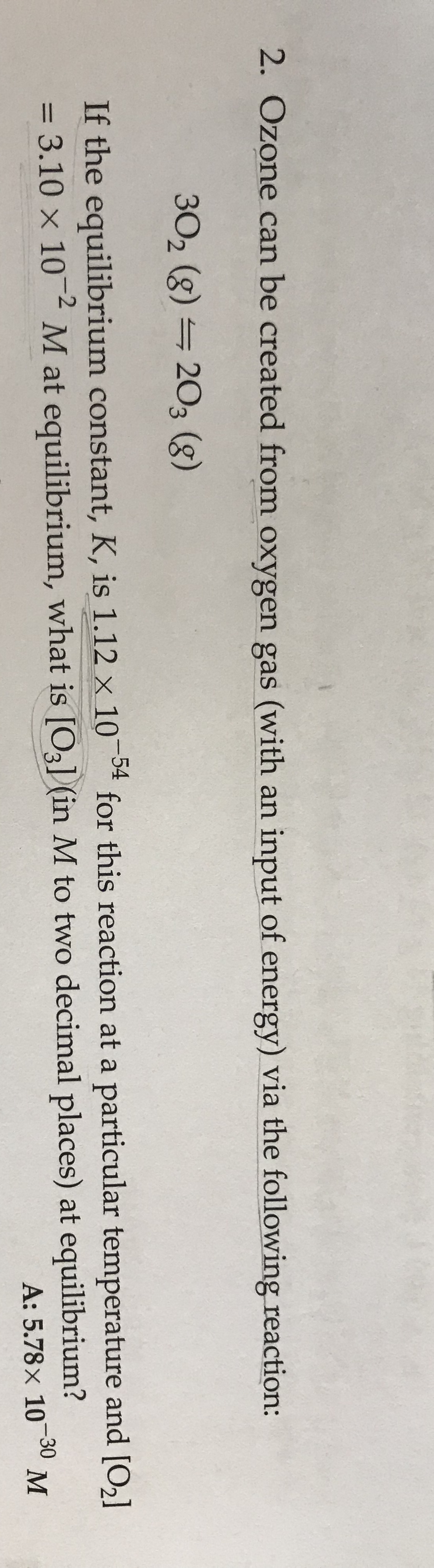# Ozone can be created from oxygen gas (with an input of energy) via the following reaction. 3O2(g) ⇌ 2O3(g) If the equilibrium constant, K, is 1.12×10^-54 for this reaction at a particular temperature and [O2] = 3.10×10^-2 M at equilibrium, what is [O3] (in M to two decimal places) at equilibrium?